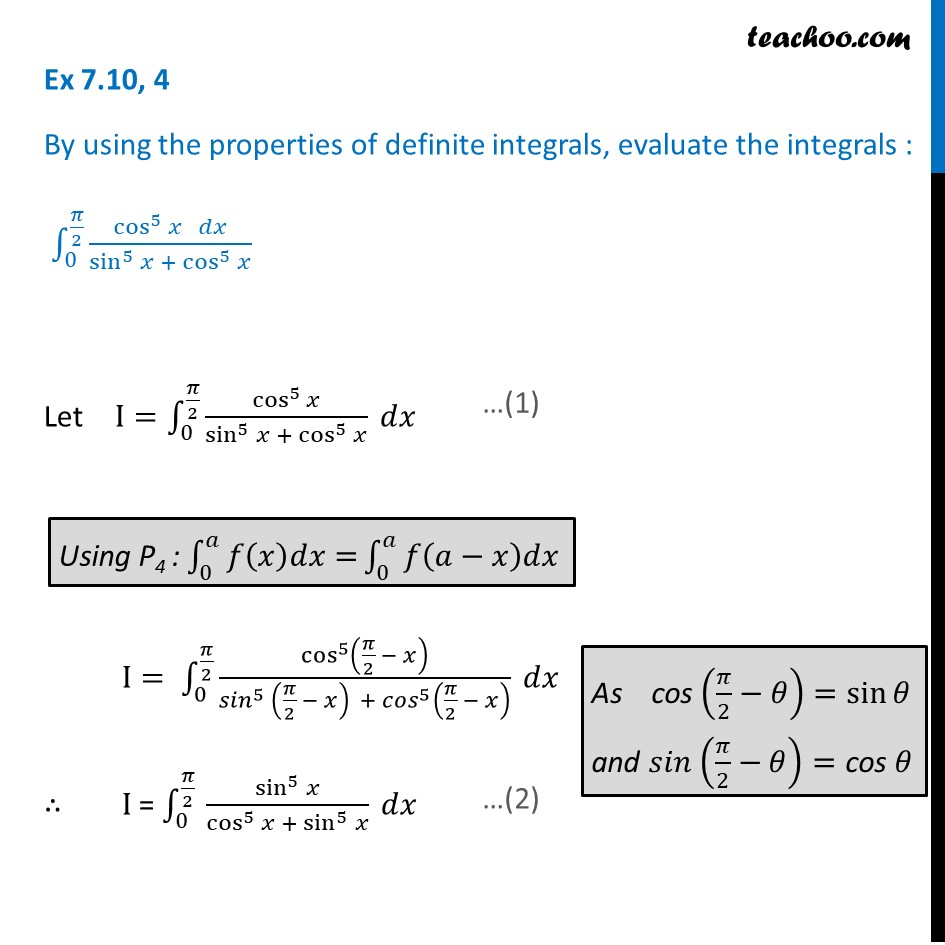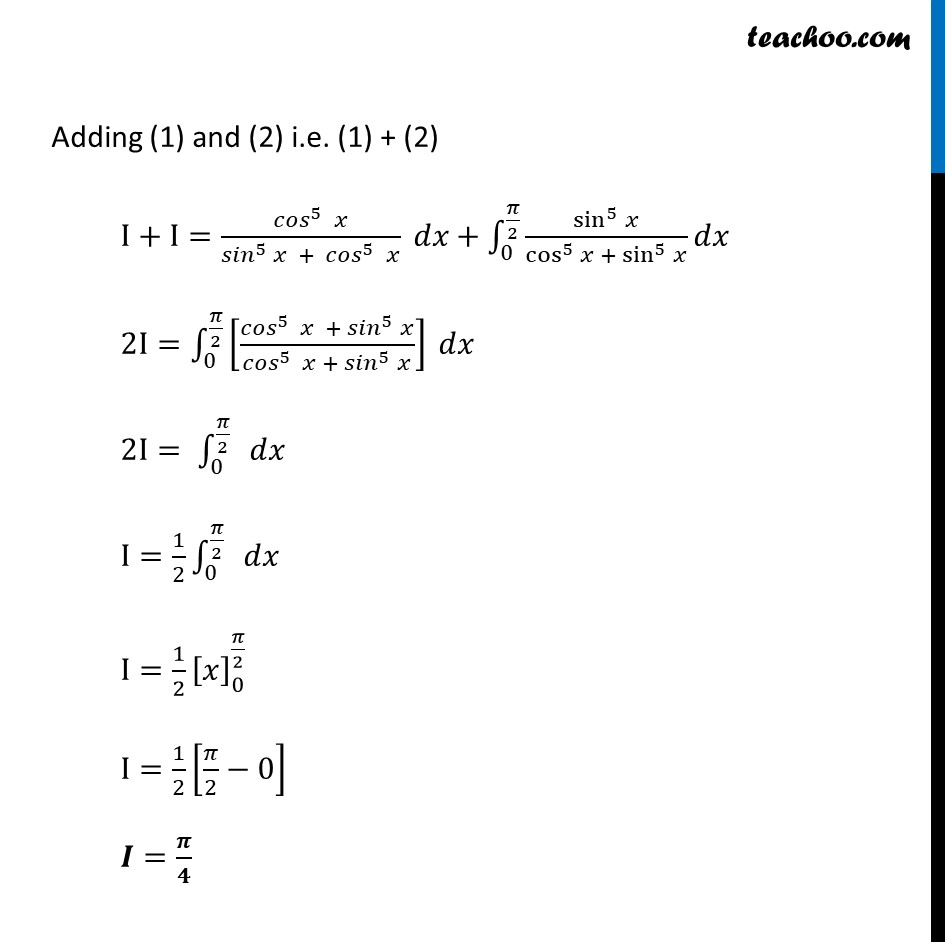Definite Integration by properties - P4

Chapter 7 Class 12 Integrals
Concept wiseLearn in your speed, with individual attention - Teachoo Maths 1-on-1 Class

### Transcript

Ex 7.10, 4 By using the properties of definite integrals, evaluate the integrals : ∫_0^(𝜋/2)▒(cos^5⁡𝑥 𝑑𝑥)/(sin^5⁡𝑥 + cos^5⁡𝑥 ) Let I=∫_0^(𝜋/2)▒〖cos^5⁡𝑥/(sin^5⁡𝑥 + cos^5⁡𝑥 ) 𝑑𝑥〗 I= ∫_0^(𝜋/2)▒〖(cos^5 (𝜋/2 − 𝑥))/(〖𝑠𝑖𝑛〗^5 (𝜋/2 − 𝑥) + 〖𝑐𝑜𝑠〗^5 (𝜋/2 − 𝑥) ) 𝑑𝑥〗 ∴ I = ∫_0^(𝜋/2)▒〖 sin^5⁡𝑥/(cos^5⁡𝑥 + sin^5⁡𝑥 ) 𝑑𝑥〗 Adding (1) and (2) i.e. (1) + (2) I+I=(〖𝑐𝑜𝑠〗^5 𝑥)/(〖𝑠𝑖𝑛〗^5 𝑥 + 〖𝑐𝑜𝑠〗^5 𝑥) 𝑑𝑥+∫_0^(𝜋/2)▒〖sin^5⁡𝑥/(cos^5⁡𝑥 + sin^5⁡𝑥 ) 𝑑𝑥〗 2I=∫_0^(𝜋/2)▒〖[(〖𝑐𝑜𝑠〗^5 𝑥 + 〖𝑠𝑖𝑛〗^5 𝑥)/(〖𝑐𝑜𝑠〗^5 𝑥 + 〖𝑠𝑖𝑛〗^5 𝑥)] 𝑑𝑥〗 2I= ∫_0^(𝜋/2)▒〖 𝑑𝑥〗 I=1/2 ∫_0^(𝜋/2)▒〖 𝑑𝑥〗 I=1/2 [𝑥]_0^(𝜋/2) I=1/2 [𝜋/2−0] 𝑰=𝝅/𝟒